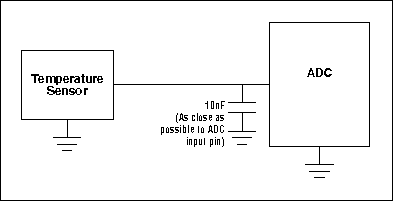# Using Analog Temperature Sensors with ADCs

### Abstract

Analog temperature sensors are often used with analog-to-digital converters (ADCs). Understanding the interface between the two devices will help the designer achieve better temperature measurement accuracy with these devices. This application note discusses the sensor-ADC interface and how to get the best results from the components used.

Analog-output temperature sensors are popular because of their low cost, small size, and low power requirements. In many systems that use analog temperature sensors, the sensor's output is measured with an analog-to-digital converter (ADC).

Understanding a few simple concepts will help you in the design of the sensor/ADC interface. In the discussion below, we will use the MAX6605, a low-power analog temperature sensor, to illustrate the concepts.

### Source Impedance

Most general-purpose ADCs in widespread use, whether discrete or embedded in a microcontroller, have a simple sample-hold circuit at their inputs. A switch connects the signal source with a sampling capacitor. After a short sampling period, the switch opens. Figure 1 shows a simplified version of an ADC input stage. Note that all ADC input stages are not the same; Figure 1 is shown as a conceptual aid only.For accurate sampling of the input signal, the capacitor must fully charge during the time that the switch is closed. For a 10-bit ADC, this means that the time constant of the total source resistance (including the switch on-resistance) multiplied by the sampling capacitance (say, 10pF) must be less than about 10% of the time that the switch is closed:

(RON + ROUT) x CSAMPLE < 0.1 x TSAMPLE

where RON is the sampling switch on-resistance, ROUT is the output resistance of the temperature sensor, CSAMPLE is the sample-hold capacitance, and TSAMPLE is time that the sampling switch is closed.

This places a constraint on the value of the source resistance driving the ADC input. Most general-purpose ADCs have maximum source-impedance limits somewhere between 1kΩ and 10kΩ.

Analog temperature sensors have output resistances that range from less than 100Ω to several kΩ; some of the higher-impedance output stages are incompatible with certain ADC inputs. The MAX6605 has a 240Ω output impedance and is compatible with virtually all general-purpose ADCs.

If a temperature sensor has excessive output resistance, connecting a capacitor between the ADC input pin and ground can reduce the effective source impedance and minimize errors. The capacitor should usually be on the order of a thousand times' the value of the sampling capacitor, so a 10nF capacitor is generally sufficient. The time between samples should be long enough to allow the capacitor voltage to approximate the sensor's output voltage to within the required accuracy. Ten time constants should be sufficient for a 10-bit ADC; therefore, for a 1k source resistance and a 10nF capacitor, a period of at least 100µs between conversions should be adequate.

Even when a capacitor isn't needed to compensate for excessive source resistance, it is common practice to place a capacitor across the ADC input pin, as shown in Figure 2. This helps to filter noise that has been picked up on the traces leading to the sensor. Common values are on the order of 1nF to 100nF. As discussed above, the sampling rate has to be slow enough to allow the capacitor to charge sufficiently between conversions.Ideally, the temperature sensor is able to drive this capacitance without oscillating. (The MAX6605 is stable with load capacitance from 1nF to infinity.) Many other analog temperature sensors become unstable when loaded with a few hundred picofarads of load capacitance; these can sometimes be isolated from the capacitance by the addition of a series resistor with a value of a few hundred ohms. Note that adding a resistor between the sensor output and the ADC input can necessitate slowing the sampling rate further.

### Reference Voltage, Temperature Range, and Resolution

The reference voltage used with the ADC affects the useful temperature range and the temperature measurement resolution. As an example, consider the MAX6605 temperature sensor driving a 10-bit ADC with a 2.5V reference. This ADC might be embedded within a microcontroller, or, in applications requiring higher performance, it might be a separate ADC such as the MAX1248.

With a 2.5V reference, each LSB will have a weight of

2.5V/1024LSBs = 2.44mV/LSB

The nominal slope of the MAX6605 temperature sensor's output is 11.9mV/°C, so each LSB will correspond to

(2.44mV/LSB)/(11.9mV/°C) = 0.205°C/LSB

The MAX6605 produces a nominal output voltage of 2.26V at 125°C; thus, its temperature and voltage ranges are compatible with the 2.5V reference.